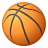# QlikView App Dev

Discussion Board for collaboration related to QlikView App Development.

Announcements
Join us at the Cloud Data and Analytics Tour! REGISTER TODAY
cancel
Showing results for
Search instead for
Did you mean:Specialist II

## Getting value of the value of a field

Hi,

I am facing a problem I can’t find the solution. You can see below part of an Excel I am using as mapping. The field Multiplier is a field I use in my expression to multiply the data by this Multiplier, for example:

=Sum(Data * Multiplier)

vDecimal is a variable I have defined in my app. The thing is that in my expression, Multiplier works if I have numeric values in my excel, as the first 3 rows, but the rows in red returns NULL. I think this is because instead of getting the value of the variable, I am getting it as a text ‘\$(vDecimal)’ in the expression and I need the value of the variable. I tried a lot of formats without success.

 Line Number Ordering Account Code Multiplier L01000 1 AAAAAAAT 1 L01010 2 BBBBBBBT 1 L01020 3 CCCCCCCT 1 L01030 4 H10000000 \$(vDecimal) L01040 5 H20000000 \$(vDecimal) L01050 6 H30000000 \$(vDecimal)

Appreciate any ideas, thanks!

Regards,

Jose

1 Solution

Accepted SolutionsContributor III

You could set blank for those lines where you want to have the multiplier variable. And in your expression do something like:

Sum(Data * alt(Multiplier, \$(vDecimal)))

2 RepliesContributor III

You could set blank for those lines where you want to have the multiplier variable. And in your expression do something like:

Sum(Data * alt(Multiplier, \$(vDecimal)))Specialist II
Author

Obrigado Guilherme! Instead of set blank those lines, I set a character 'N'